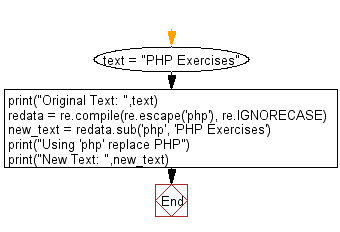﻿ Python: Do a case insensitive string replacement - w3resource# Python: Do a case-insensitive string replacement

## Python Regular Expression: Exercise-44 with Solution

Write a Python program to do a case-insensitive string replacement.

Sample Solution:-

Python Code:

``````import re
text = "PHP Exercises"
print("Original Text: ",text)
redata = re.compile(re.escape('php'), re.IGNORECASE)
new_text = redata.sub('php', 'PHP Exercises')
print("Using 'php' replace PHP")
print("New Text: ",new_text)
```
```

Sample Output:

```Original Text:  PHP Exercises
Using 'php' replace PHP
New Text:  php Exercises
```

Pictorial Presentation:Flowchart:## Visualize Python code execution:

The following tool visualize what the computer is doing step-by-step as it executes the said program:

Python Code Editor:

Have another way to solve this solution? Contribute your code (and comments) through Disqus.

What is the difficulty level of this exercise?

Test your Programming skills with w3resource's quiz.

﻿

## Python: Tips of the Day

Combining two iterable of tuples or pivot nested iterables:

```# Combining two iterables
>>> a = [1, 2, 3]
>>> b = ['a', 'b', 'c']
>>> z = zip(a, b)
>>> z
[(1, 'a'), (2, 'b'), (3, 'c')]

# Pivoting list of tuples
>>> zip(*z)
[(1, 2, 3), ('a', 'b', 'c')]
```